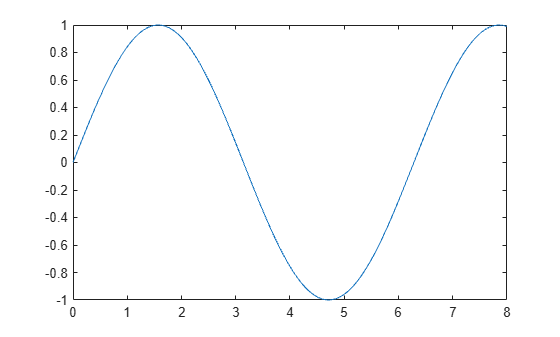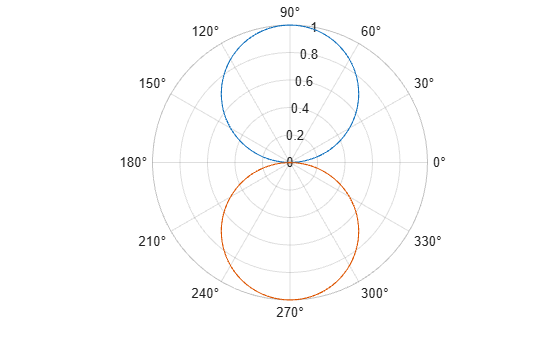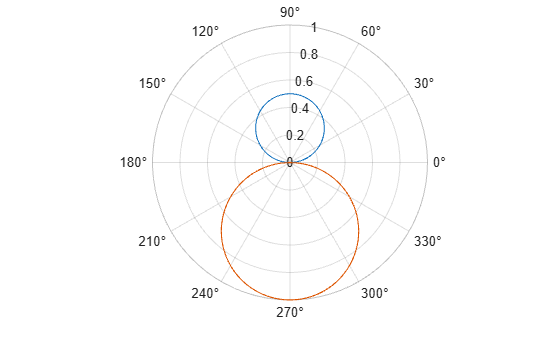# refreshdata

Refresh charted data

## Syntax

``refreshdata``
``refreshdata(target)``
``refreshdata(target,workspace)``

## Description

example

````refreshdata` updates charted data in the current figure to reflect changes in workspace variables. Before using `refreshdata`, you must first set the data source properties (for example, `XDataSource`) of the charts. The function evaluates these data sources before updating the charts.```

example

````refreshdata(target)` updates charted data in the specified figure, axes, or chart with data source properties.```
````refreshdata(target,workspace)` specifies the workspace where the data source properties are evaluated.```

## Examples

collapse all

Refresh charted data to reflect changes in workspace variables.

First, plot some data and return a `Line` object.

```x = linspace(0,8); y = sin(x); ln = plot(x,y);```Identify the data sources for the chart by setting the `XDataSource` and `YDataSource` properties of the `Line` object. Then, modify `y`. Update the chart by calling `refreshdata`.

```ln.XDataSource = 'x'; ln.YDataSource = 'y'; y = sin(3.*x); refreshdata```Refresh charted data for a specific line and leave other lines unchanged.

First, plot two sets of polar data and return the `Line` objects.

```theta = linspace(0,2*pi); rho1 = sin(theta); rho2 = -sin(theta); px = polaraxes; hold on; ln1 = polarplot(theta,rho1); ln2 = polarplot(theta,rho2);```Set the `RDataSource` property for each line. Then, modify `rho1` and `rho2`.

```ln1.RDataSource = 'rho1'; ln2.RDataSource = 'rho2'; rho1 = 0.5*sin(theta); rho2 = 0.5*cos(theta);```

Update only the first line by using `ln1` as the input argument to `refreshdata`. Note that the second line remains unchanged.

`refreshdata(ln1)`## Input Arguments

collapse all

Target object, specified as a `Figure` object, `Axes` object, `PolarAxes` object, `GeographicAxes` object, or chart object with data source properties. For example, `Line` objects have an `XDataSource` and `YDataSource` property.

Workspace where data source properties are evaluated, specified as one of these values:

• `'base'` — Evaluate data source properties in the base workspace.

• `'caller'` — Evaluate data source properties in the workspace of the function that called `refreshdata`.

If you set data source properties of variables that are not in the base workspace, then you must specify the workspace option as `'caller'`.

## Version History

Introduced before R2006a